• 概率分析与随机算法 <说明> 该文章内容我尚未理解深刻，此处仅作记录 文章目录概率分析与随机算法1.雇用问题1.1概率分析、平均情况运行时间 1.雇用问题 假如你要雇用一名新的办公助理。你先前的雇用尝试都失败...
概率分析与随机算法
<说明>
该文章内容我尚未理解深刻，此处仅作记录
文章目录概率分析与随机算法1.雇用问题1.1概率分析、平均情况运行时间1.2随机算法、期望运行时间2.随机算法（随机排列数组）2.1 PERMUTE-BY-SORTING(优先级）2.2 RANDOMIZE-IN-PLACE(位置）3 概率分析3.1 生日悖论（使用期望、多次积分）3.2 球与箱子、礼券收集者问题
1.雇用问题
假如你要雇用一名新的办公助理。你先前的雇用尝试都失败了，于是你决定找一个雇用代理。
·雇用代理每天给你推荐一个应聘者。
·你面试这个人，然后决定是否雇用他。
（你必须付给雇用代理一小笔费用，以便面试应聘者；然而真正雇用一个应聘者需要花更多的钱，因为你必须辞掉目前的办公助理，还要付一大笔中介费给雇用代理）
·你承诺任何时候，都要找最适合的人来担任该项职务。（即在面试完每个应聘者后，如果该应聘者比目前的办公助理更合适，就会辞掉当前的办公助理，然后聘用新的。）
~你希望能够估算该费用会是多少？
-伪代码展示：
（假设应聘办公助理的候选人编号为1到n。该过程假设你能在面试完应聘者i后，决定应聘者i是否是你目前见过的最佳应聘者。初始化时，该过程创建一个虚拟的应聘者，编号为0，他比其他应聘者都差）
HIRE-ASSISTANT(n)
1	best=0  // candidate 0 is a least-qualified dummy candidate
2	for i=1 to n
3		interview candidate i
4		if candidate i is better than candidate best
5			best=i
6			hire candidate i

//感觉是在找出数组中的最大数,不过求得内容不同
//伪代码用python写还不错哈哈
//在任何情形中，我们都是计算特定基本操作的执行次数。
分析：
假设面试一个人的费用是a，雇用一个人的费用是b，显然b>a恒成立。
在问题假设中，一共面试n个人，所以面试产生的费用是固定的即an,
假设此次面试中共雇用了m个人，雇用产生的费用为bm，所以可以说，估算总费用取决于m变量。
提升：
这个场景用来作为一般计算范式的模型。
我们通常通过检查序列中的每个成员，并且维护一个当前的 “获胜者” ，来找出序列中的最大值或最小值。这个雇用问题对当前获胜成员的更新频率建立模型。
最坏情况分析： 每次面试都需要雇用，即面试n次，雇用了n次，总的费用是O(bn)……因为b>a……
但事实上，我们并不知道应聘者出现的次序，最坏情况出现的概率并不多。但我们想知道在一种自然情况或者平均的情形下， 会有什么发生，这时的总费用是多少。
平均情况分析： 实际情况下，由于该公司的应聘策略是，如果该应聘者比之前的应聘者都优秀，则应聘他。所以，对于第i个应聘者来说，应聘成功的概率也就是1/i；用Xi表示第i个应聘者是否应聘成功,则E(Xi)=1/i。设该公司在面试n个应聘者后总的应聘次数为X=X1+X2+…+Xn
所以该公司在面试n个应聘者后总的应聘次数的期望为
$E(X)= E \left[\LARGE{\displaystyle\sum_{i=1}^nX_i }\right] = \displaystyle\sum_{i=1}^nE(X_i)=\displaystyle\sum_{i=1}^n1/i=\ln n + O(1)$
所以说平均情况下，算法HIRE-ASSISTANT的总的雇用费用为O(blnn).
1.1概率分析、平均情况运行时间
概率分析是在问题分析中应用概率的理念。大多数情况下，我们采用概率分析来分析一个算法的运行时间，有时也用它来分析一些其他的量。
为了使用概率分析，我们需要使用或者假设关于输入的分布，然后分析该算法，计算出一个平均情形下的运行时间，其中，我们对所有可能的输入分布产生的运行时间取平均值。称为平均情况运行时间。
1.2随机算法、期望运行时间
一般地，如果一个算法的行为不仅由输入决定，而且也由随机数生成器产生的数值决定，则称这个算法是随机的。
当分析一个随机算法的运行时间时，我们以运行时间的期望值衡量，其中输入值由随机数生成器产生。我们将一个随机算法的运行时间称为期望运行时间。

一般而言，当概率分布是在是算法的输入上时，我们讨论的是平均情况运行时间；当算法本身做出随机选择时，我们讨论其期望运行时间。
2.随机算法（随机排列数组）
2.1 PERMUTE-BY-SORTING(优先级）
伪代码如下：
PERMUTE-BY-SORTING(A)
1	n=A.length
2	let P[1..n] be a new array
3	for i=1 to n
4		P[i] = RANDOM(1,n^3)
5	sort A, using P as sort keys


使用范围1~n^3是为了让P中所有优先级都尽可能唯一
那如何在多优先级相同的情况下实现这个算法呢？
笔者认为：只需要改一下排序时的比较条件就可以满足，such as : 将大于改为大于等于。
同时需要证明该过程能产生一个均匀随机数列（即该过程等可能地产生了数字1~n的每一种排列）————证明过程略去。

2.2 RANDOMIZE-IN-PLACE(位置）
伪代码如下：
RANDOMIZE-IN-PLACE(A)
1	n=A.length
2	for i=1 to n
3		swap A[i] with A[RANDOM(i,n)]


需要证明该过程能产生一个均匀随机数列 ————证明过程略去

两种算法相比较，后者更好（时间复杂度、空间复杂度）
3 概率分析
3.1 生日悖论（使用期望、多次积分）
问题描述：一个屋子里人数必须要达到多少人，才能使其中两人的生日相同的机会达到50%？
使用指示变量进行分析如下：
对屋子里k个人中的每一对(i,j)，对1<=i<=j<=k，定义指示变量Xij如下
$X_{ij}=I(i和j生日相同)= \begin{cases} 1 &\text{if } {i和j的生日相同} \\ 0 &\text{else } \end{cases}$

两个人i和j的生日正好相同的概率依赖于生日的选择是否独立。

假设生日是独立的。

设一年有n天，则一个人出生在每一天的概率均为1/n，于是i和j出生在同一天r的概率为
$Pr(b_i=r 且 b_j = r)=Pr(b_i=r)Pr(b_j=r)=1/n^2$

这样他们的生日落在同一天的概率为
$Pr(b_i=b_j)=\displaystyle\sum_{r=1}^nPr(b_i=r 且 b_j = r)=\displaystyle\sum_{r=1}^n(1/n^2)=1/n$
(这个计算最后使用到了积分和级数）

继而有
$E\begin{bmatrix} X_{ij} \end{bmatrix}=Pr(i和j生日相同)=1/n$
(0-1分布)

设X 表示计数生日相同两人对数目的随机变量，则有：
$X=\displaystyle\sum_{i=1}^k\displaystyle\sum_{j=i+1}^kX_{ij}$

两边取期望，并应用期望的线性性质，有：
$E\begin{bmatrix} \displaystyle\sum_{i=1}^k\displaystyle\sum_{j=i+1}^kX_{ij} \end{bmatrix}= \displaystyle\sum_{i=1}^k\displaystyle\sum_{j=i+1}^kE\begin{bmatrix} X_{ij} \end{bmatrix}= \begin{pmatrix} k \\ 2 \end{pmatrix}1/n=k(k-1)/2n$

因此，当k(k-1)>=2n时，生日相同的两人对的期望数至少是1.也就是说， 若屋子里至少有√2n+1个人，我们可以期望至少有两人生日相同。

3.2 球与箱子、礼券收集者问题
一个人如果想要收集齐b种不同礼券中的每一种，大约需要blnb张随机的到的礼券。
分析如下：

可以把集齐b种分为b个阶段，一个阶段集齐一种。第i个阶段包括从第i-1次命中到第i-1次命中之间的投球。
第1阶段包含第一次投球，因为我们可以一次保证命中，此时所有的箱子都是空的。对第i阶段的每一次投球，有i-1个箱子有球，b-i+1个箱子是没有球的，所有，对于第i阶段的每次投球，得到一次命中的概率为(b-i+1)/b.
设ni表示第i阶段的投球次数。每个随机变量ni服从几何分布，成功的概率是(b-i+1)/b，由几何分布的期望可知，有：
$E\begin{bmatrix} n_{i} \end{bmatrix}={b \over b-i+1}$
为得到b次命中所需的投球数n=n1+n2+…+nb
由期望的线性性质可知：
$E\begin{bmatrix} n \end{bmatrix}=E\begin{bmatrix} \displaystyle\sum_{i=1}^b n_{i} \end{bmatrix}=\displaystyle\sum_{i=1}^bE\begin{bmatrix} n_{i} \end{bmatrix}=\displaystyle\sum_{i=1}^b{b \over b-i+1}=b\displaystyle\sum_{i=1}^b{1 \over i}=b(\ln b+O(1))$


展开全文• 数组的随机排列算法及其简单验证1.1 数组的排列的随机算法的最终目的是达到，数组每个元素在每个位置上出现的概率为1/n（其中n为数组大小）。一种的算法如下，对于数组A中的每个元素A[i]，随机附上一个权值，然后...

1. 数组的随机排列算法及其简单验证2.随机算法简单分析3. 代码下载  1.  数组的随机排列算法及其简单验证1.1 数组的排列的随机算法的最终目的是达到，数组每个元素在每个位置上出现的概率为1/n（其中n为数组大小）。一种的算法如下，对于数组A中的每个元素A[i]，随机附上一个权值，然后根据该权值对A数组进行排序。下面是一个简单的实现，排序使用的是冒泡排序，代码如下：        public static void PermuteBySorting(ref int[] A)        {            int length = A.Length;            List<int> B =                 new List<int>();            // 随机数            System.Random rand = new System.Random();            foreach (int item in A)            {                // [1, length^3]                B.Add(rand.Next(1, (int)Math.Pow(length, 3)));            }            // 根据key排序，这里使用冒泡排序            int tmp;            for (int i = 0; i < B.Count; ++i)                for (int j = B.Count - 1; j >= (i + 1); --j)                {                    // 递增，交换数据                    if(B[i] > B[j])                    {                        // 没有必要记录A中的数据，交换A中的数据                        tmp = A[i];                        A[i] = A[j];                        A[j] = tmp;                    }                }        }1.2 另外一种数组随机算法如下：遍历数组，随机选择数组的另外的一个元素，交换这两个元素的位置。算法比较简单，实现代码如下：// 就地排序        public static void RandomizeInPlace(ref int[] A)        {            int length = A.Length;            System.Random rand = new System.Random();            int num, tmp;            for (int i = 0; i < length; ++i )            {                num = rand.Next(0, (length - 1));                tmp = A[i];                A[i] = A[num];                A[num] = tmp;            }            // 输出结果             foreach(int item in A)            {                Console.WriteLine(item);            }	  }        2. 随机算法分析随机算法中一种比较重要的分析方法是使用指示器分析法。定义变量如下：定义样本空间S事件A 随机变量指示器II(A)定义如下：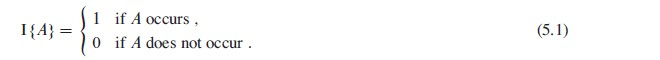一个简单的使用随机指示器变量的例子：我们都知道如果硬币是均匀的话，投掷硬币n次出现正面的可能次数是n/2，也就是说正面的出现次数和反面出现的次数在大量的统计上是相同的，下面我们从数学的方法去证明这个事实。 定义随机变量X表示n次掷硬币出现的正面的次数，该事件能够分解成如下的Xi，表示第i次投掷硬币出现正面向上的事件。于是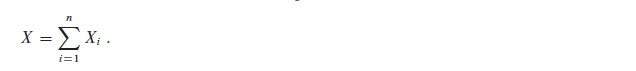两边取期望值：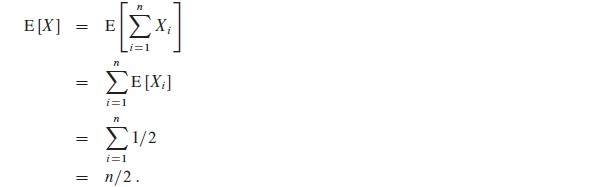即证明n次投掷硬币的工程中，可能出现正面朝上的次数是n/2。通过上面的例子的分析可以看出最主要的是定义事件X，然后将事件X分解成较小子事件Xi，最后求得X的期望值。利用上面的方法同样呢能够证明“生日悖论”等问题。3.代码下载/Files/xuqiang/Random.rar

转载于:https://www.cnblogs.com/xuqiang/archive/2011/03/16/1985977.html
展开全文• * * * * * * * 5.3.2 Randomly permuting an array (2) RANDOMIZE-IN-PLACE RANDOMIZE-IN-PLACE(A, n) for(i=1; i; i++) swap(A[i], A[RANDOM(i, n)] Lemma RANDOMIZE-IN-PLACE computes a uniform random permut
• 练习5.2-2 问题描述：在 HIRE-ASSISTANT中，假设应聘者以随机的顺序出现，正好雇佣两次的概率是多少？分析：要想正好雇佣两次，首先要考虑三个条件：1. 候选人i总是被雇佣2. 最佳候选人，也即候选人n也被雇佣3. 若...
练习5.2-2 问题描述：在 HIRE-ASSISTANT中，假设应聘者以随机的顺序出现，正好雇佣两次的概率是多少？分析：要想正好雇佣两次，首先要考虑三个条件：1. 候选人i总是被雇佣2. 最佳候选人，也即候选人n也被雇佣3. 若最佳候选人是i，则i是唯一的候选人因此，候选人i的顺序要满足i <= n – 1且所有排序在候选人n之前的i + 1，i + 2，…，n – 1的候选人都被面试了。令事件表示候选人i被雇佣，则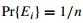对任意i。令事件F表示在事件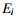发生后，最佳候选人从n-i中的i + 1，i + 2，…，n – 1，n中出现。因此，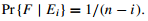则事件A即刚好雇佣两次出现的情况是：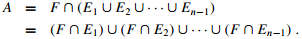事件A出现的概率为：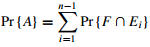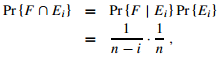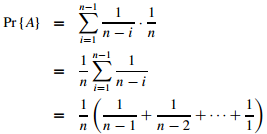练习5.2-5 问题描述：假设A[1..n]是由n个不同的数构成的数组。如果i < j且A[i] > A[j]，则称(i, j)为逆序对。假设A的元素为1到n的随机排列。利用指示器随机变量来计算A中逆序对的期望数目。设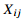为指示器随机变量，用来表示逆序对事件的出现，定义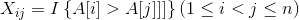，则任意给定两个数，较小数出现较大数之前的概率为，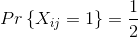，则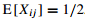。令随机变量X表示总的逆序数目，则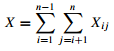根据期望的线性不变性：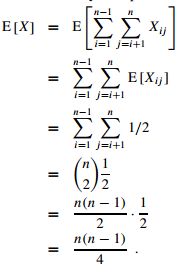因此，逆序对数目的期望值为n(n - 1) / 45.3随机算法随机算法通过排列给定的输入数组来使输入随机化。下面讨论两种随机化方法： 1. 优先级随机化排序PERMUTE-BY-SORTING   思想：为数组A的每个元素A[i]赋一个随机的优先级P[i]，然后依据优先级对数组A中的元素进行排序。例如，如果初始数组A=[1,2, 3, 4]且选择随机的优先级P =[36, 3, 97, 19]，则将根据指定的优先级得到数列B=[2,4, 1, 3]。这个过程伪代码为：PERMUTE-BY-SORTING(A)n ← length(A)for i ←1 to nP[i]= RANDOM(1, n^3)sort A,using P as sort keysreturn A第三行选取1到n^3之间的随机数，为了让P中的所有优先级尽可能是唯一的。此过程的时间复杂度主要体现在第4行中的排序将花费O(nlgn)，如果P[i]是第j个最小的优先级，那么A[i]将在输出位置j上。下面来证明这个过程能产生均匀的随机排列，即数字1到n的每一种排列都是等可能被产生。证明：考虑每个元素A[i]得到第i个最小优先级的特殊排列开始，并证明这个排列发生的概率正好是1/n!。对于i=1,2,…,n，令Xi代表元素代表元素A[i]得到第i个最小优先级的事件，比如A得到的第1个最小优先级。则对所有i，事件Xi发生的概率为因为Pr{X1}是从n个元素的集合中随机选取优先级是最小的概率，故Pr{X1}=1/n。接下来有Pr{X2|X1}=1/(n-1)，由于假定A有最小的优先级，则余下的n-1个元素有相等的成为第2个优先级的可能。一般地对于i=2,3, …, n有：$\Pr \{ {X_i}|{X_{i - 1}} \cap {X_{i - 2}} \cap \cdot \cdot \cdot \cap {X_1}\} = 1/(n - i + 1)$这是因为从A到A[i-1]按顺序有前i-1小的优先级，余下的n-(i-1)中，每个具有第i个小优先级的可能性都相同。所以有：$\Pr \{ {X_1} \cap {X_2} \cap {X_3} \cap ... \cap {X_{n - 1}} \cap {X_n}\} = \left( {\frac{1}{n}} \right)\left( {\frac{1}{{n - 1}}} \right) \cdot \cdot \cdot \left( {\frac{1}{2}} \right)\left( {\frac{1}{1}} \right) = \frac{1}{{n!}}$这样就证明了得到同样排列的概率是1/n!。继续扩展这个证明，使其对任何优先级的排列都起作用。考虑集合{1,2, …, n}的任意一个确定的排列S=[s(1), s(2),…, s(n)]。用Ri代表赋予元素A[i]的优先级排名，其中第j小的元素的优先级名次为j。如果定义Xi为元素A[i]的得到第s(i)优先级的事件，或者Ri=s(i)，那么同样的证明仍然适用。因此，如果要计算得到任何特殊排列的概率，这个计算和先前的计算完全相同，所以得到这个排列的概率也是1/n!。 2. 原地排列给定数列的随机排列法原地随机抽取元素法，即在第i次迭代时，元素A[i]是从元素A[i]到A[n]中随机选取的。第i次迭代之后，A[i]保持不变。程序RANDOMIZE-IN-PLACE在O(n)时间内完成。伪代码如下：RANDOMIZE-IN-PLACE(A)n ←length(A)for i ←1 to nswapA[i] ↔ A[RANDOM(i, n)]下面用循环不变式证明程序RANDOMIZE-IN-PLACE能产生均匀随机排列。给定包含n个元素的一个集合，k排列是包含这n个元素k个元素的序列，共有n!/(n-k)!种可能的k排列。这是由于给定k个位置，从n个元素选元素，第一位置的可能性为n，接着为n-1，…，直到最后一个位置有n-k+1个可能性。所以共有：$n \cdot (n - 1) \cdot (n - 2) \cdot \cdot \cdot (n - k + 1) = \frac{{n!}}{{(n - k)!}}$种可能的k排列。证明：使用循环不变式：在第2-3行for循环的第i次迭代之前，对每个可能(i-1)排列，子数列A[1...i-1]包含这个(i-1)排列的概率为(n– i + 1)! / n!。需要证明这个不变式在第一次迭代之前为真，循环的每次迭代能够保持此不变式，并且在循环结束时，次不变式提供一个有用的属性来证明循环结束时的正确性。初始化：考虑第一次循环迭代之前的情况，i=1。由循环不变式知，对每个可能的0排列，子数组A[1..0]包含这个0排列的概率为(n– i + 1)! / n! = 1。子数列A[1..0]是空的子数组，且0排列也没有任何元素。所以A[1..0]包含所有0排列的概率为1，在第1次循环迭代之前循环不变式成立。保持：假设刚好在第i次迭代之前，每种可能的(i-1)排列出现在子数组A[1..i-1]中的概率是(n – i + 1)! / n!，要证明在第i次迭代之后，每种可能的i排列出现在子数组A[1..i]的概率是(n– i )! / n!。考虑一个特殊的i排列，以[x(1),x(2), …, x(i)]来表示其中的元素。这个排列包含一个(i-1)排列[x(1), x(2),…, x(i-1)]，接着算法在A[i]中放置的值x(i)的事件用E1表示，它表示前i-1跌代已经在A[1…i-1]构造了此特殊的(i-1)排列。由循环不变式，Pr{E1}=(n– i + 1)! / n!。用E2表示第i次迭代在A[i]中放置值x(i)的事件。E1和E2都发生时，则次特殊的i排列形成。此时概率有：$\Pr \{ {E_2} \cap {E_1}\} = \Pr \{ {E_2}|{E_1}\} \Pr \{ {E_1}\}$概率Pr{E2| E1}等于1/ (n – i + 1)，因为算法要从位置A[i..n]的n-i+1个值中随机选取一个值命为x(i)。因此有：$\Pr \{ {E_2} \cap {E_1}\} = \Pr \{ {E_2}|{E_1}\} \Pr \{ {E_1}\} = \frac{1}{{n - i + 1}}\frac{{(n - i + 1)!}}{{n!}} = \frac{{(n - i)!}}{{n!}}$终止：在结束时，i=n+1，的子数组A[1..n]是一个给定n排列的概率为(n- n)! / n! = 1 / n!。因此，RANDOMIZE-IN-PLACE是可以计算出一个均匀随机排列的。随机算法通常是解决问题的最简单也是最有效的方法。
展开全文• 知识点总结 概率分析是在问题分析中应用概率的理念，必须要假设输入的分布，然后...当分析一个随机算法的运行时间时，我们以运行时间的期望值来衡量，其中输入值由随机数生成器产生，我们称为期望运行时间。 当我们在概
知识点总结

概率分析是在问题分析中应用概率的理念，必须要假设输入的分布，然后计算出一个平均情形下的运行时间，其中对所有可能的输入分布取平均值，得到的这种运行时间称为平均情况运行时间
如果一个算法的行为不仅由输入决定，而且也由随机数生成器产生的数值决定，我们称这个算法是随机的。当分析一个随机算法的运行时间时，我们以运行时间的期望值来衡量，其中输入值由随机数生成器产生，我们称为期望运行时间。
当我们在概率分析时，是假设输入具有某种分布。但是在分析随机算法时，我们能通过该算法内部的随机数生成器产生我们想要的输入分布。也就是说在概率分析时，具有某种输入的分布是虚拟的，是人为假设的；但是对于随机算法来说，具有某种输入的分布是真真实实的，可以人为得到的。因此为了便于区分，我们将概率分析时得到的平均时间称之为平均情况运行时间，但是对于随机算法来说，我们将得到的平均时间称之为期望运行时间，有点细微差别。
指示器随机变量： 给定一个样本空间S和一个事件A，那么事件A对应的指示器随机变量 I{A} 定义为：I{A}=1（如果A发生）；I{A}=0 （如果A不发生）。因此I{A}的期望值E[I{A}]=1*Pr{A发生}+0 *Pr{A不发生}=Pr{A发生}。具体应用例子可以看5.2-4和5.2-5
算法或例子代码

我列举了随机数组排列，周期循环的随机数组排列和数组中一个子集的随机排列这三种算法代码。

随机数组排列：
~~~c++
#include
#include
using namespace std;

template<class Type>
void randomPermuteArray(vector<Type>& array)
{
if(array.size()==0){
cout<<"The array is empty?!"<<endl;
return;
}

for(int i=0;i!=array.size();++i)
{
// random number in the range between i and the end of the array
int randomPosition=rand()%(array.size()-i)+i;
swap(array[i],array[randomPosition]);
}
}


~~~

该算法可以理解为在箱子里面有n个不同的小球，现在从箱子里面不放回的拿小球，一共有 n!<!--//--><![CDATA[//><!--
n!
//--><!]]> 中取法，那么取到一种特定的n个球的组合概率为 1/(n!)<!--//--><![CDATA[//><!--
1/(n!)
//--><!]]>。

周期循环的随机数组排列
假设有一个数组为{1,2,3,4,5}，周期循环的随机数组可以为{2,3,4,5,1}，{3,4,5,1,2}等等，也就是说原数组中每一个元素偏移一个固定的量，但这固定的量是随机的。

#include <vector>
#include <cstdlib>
using namespace std;

template<class Type>
void cyclicRandomPermuateArray(const vector<Type>& array,vector<Type>& resultArray)
{
if(array.size()==0){
cout<<"The array is empty()?!"<<endl;
return;
}

resultArray.clear();
resultArray.resize(array.size());
// to porduce a random offset
int offset=rand()%array.size();

for(int i=0;i!=array.size();++i)
{
int dest=offset+i;
if(dest>array.size()-1)
dest-=array.size();
resultArray[dest]=array[i];
}
}


数组中一个子集的随机排列
假设有一个n维数组，现在不需要产生整个数组的随机排列，而只是想要产生其中m(m

#include <vector>
#include <cstdlib>
using namespace std

bool isBelong(const Type& val,const vector<Type>& array)
{
if(array.size()==0)
return false;

for(int i=0;i!=array.size();++i)
if(val==array[i])
return true;

return false;
}
template<class Type>
void randomSubArrayRec(int subsetNumber,int rightEnd,vector<Type>& resultSubArray)
{
if(subsetNumber==0)
return;

randomSubArrayRec(subsetNumber-1,rightEnd-1,
resultSubArray);
int randomPosition=rand()%(rightEnd+1);

if(isBelong(randomPosition,resultSubArray)) // the elements in array have to be different from each other.
resultSubArray.push_back(rightEnd);
else
resultSubArray.push_back(randomPosition);
}

template<class Type>
void randomSubArray(int subsetNumber,const vector<Type>& array, vector<Type>& resultSubArray)
{
if(array.size()==0){
cout<<"The array is empty?!"<<endl;
return;
}

if(subsetNumber>array.size()){
cout<<"Please verify the number of subset!"<<endl;
return;
}

vector<int> randomArrayPosition;
// a random subset of element positions not element itself!
randomSubArrayRec(subsetNumber,array.size()-1,
randomArrayPosition);

resultSubArray.clear();
for(int i=0;i!=subsetNumber;++i)
resultSubArray.push_back(array[randomArrayPosition[i]]);
}


在上面的算法中，我们首先产生了元素位置的随机子集，而不是直接产生元素本身的随机子集，因此上述程序第26行要求用于产生随机子集的数组不能有相同的元素。既然产生了元素位置的随机集合并且元素位置对应一个元素，因此也就产生了一个子集的随机元素集合。
展开全文• 目录雇用问题描述概率分析随机算法指示器随机变量雇用问题指示器 雇用问题描述 一个老板想换掉自己的办公助理，并且决定每次面试一个人，如果当前这个面试者比现在的办公助理好，就雇用他，并且解聘现在的办公助理...
• 概率与计算：随机算法与概率分析（Michael Mitzenmacher等著，英文扫描版）： 　本书详细地介绍了概率技术以及在概率算法与分析发展中使用过的范例。本书分两部分，第一部分介绍了随机抽样、期望、马尔可夫不等式、...
• 中国科学技术大学 算法导论 课件 计算机相关专业必修
• 假设剑的数量一定并且你在游戏过程中都会碰到，而且每次哪把剑出现是随机的，问你要付金币数的期望。  很显然，付金币的数量决定于剑出现的顺序。一般游戏中都会先出现低级的剑，所以你付的金币数会很多
• 非常好的随机算法设计与分析的书籍，讲解随机算法中的数学知识《概率与计算》
• 第5章 概率分析和随机算法本章通过四个例子来理解概率分析和随机算法。5.1 生日悖论 5.2 球箱子 5.3 特征序列 5.4 在线雇佣问题
• 概率分析与随机随机算法： 在雇佣问题中，如果应聘者是以随机顺序出现的话，雇佣一个新的办公室助理的期望次数是lnn。这个算法是随着输入的变化而变化的。对于某个特定的输入，它总是会产生固定的雇佣次数。...
• 假设A中元素形成上的一个均匀随机排列，利用指示器随机变量来计算A中逆序对的期望。 分析：题目要求这n个数是不同的数，因此A[i]要么大于A[j]要么小于A[j]，即A[i]>A[j]的概率为1/2。计算一个数组的逆序对可以这么来...
• 概率算法;随机数;数值概率算法 ;用随机投点法计算?值;拉斯维加斯( Las Vegas )算法;n后问题;蒙特卡罗(Monte Carlo)算法;蒙特卡罗(Monte Carlo)算法;素数测试;主元素问题;近似算法;近似算法;近似算法的性能; 子集合...
•  把点随机打乱之后， 先取两个点，初始化圆，然后继续加点， 如果在当前圆的外面，那么————》这个点一定在“更新圆”上，那么问题转化为确定一个点，求一个圆覆盖，递归后继续做，同样问题可以转化为确定两个点...include struct c
• 《目录》 前置知识 雇用问题 随机 期望时间 随机排布数组 洗牌算法 ...【随机变量】：在郑骰子等与概率有关的问题中，我们想要定量的思考这些问题，就需要一个叫“随机变量”的武器。 就...
• 概率分析：是将所有可能输入的运行时间作平均，对于给定的输入，其返回结果...随机算法必须保证对输入的重排能够产生均匀的随机排列，每一种排列的可能性初始输入的分布概率一致。 下一步学习学习随机算法的应用...
• 蒙特卡罗(Monte Carlo)算法并不是一种特定的算法，而是对一类随机算法的特性的概括。它的名字来源于赌城蒙特卡罗，象征概率。它的基本思想是通过大量随机样本，去了解一个系统，进而得到要计算的值。它非常强大灵活...
• 为提高图像缩放的速度，提出一种结合阈值学习概率随机裁剪的快速内容感知图像缩放算法，通过计算图像的重要度图，利用径向基函数（RBF,radial basis function）神经网络进行阈值学习求出图像的重要度阈值，根据...径向基函数
• 在极大似然估计中，我们就是用求最值的方法，将使得p(x∣θ)p(x|\theta)p(x∣θ)取得最大值的参数θ\thetaθ作为我们的估计值，有一类概率模型比较简单，他只有观测变量xxx，就像是我们在第一讲里介绍的单中心的高斯...机器学习 python 人工智能 java
• 第七章 随机算法及 NP 完全问题 ? 7.1 随机算法引言 ? 7.2 随机算法的类型 ? 7.3 随机数发生器 ? 7.4 数值概率算法 ? 7.5 舍伍德 (Sherwood) 算法 ? 7.6 拉斯维加斯 Las Vegas 算法 ? 7.7 蒙特卡罗 Monte Carlo 算法...
• 1 球箱子问题（礼券收集者问题）： 有b个箱子，每投一次球，球等可能地落到每个箱子中，问，投多少次球，才能使每个箱子都至少有一个球？ 【补充知识】 ① 几何分布的概念：假定我们有一系列伯努利试验，其中...
• 读书笔记正文下载（PDF）：http://download.csdn.net/source/504404 这个章节的内容说难不难，说简单也不简单，要是有一点概率基础的话看懂还是挺容易的，但是深究下去的话是有一定难度的。考虑到自己的当前进度...
• 在重新做《复杂》一书中第九章提到的遗传算法例子的时候遇到了一个问题，遗传算法驱动的机器人罗比需要在不断的进化过程中产生出可以清理10X10方格内随机位置垃圾的最优策略。 10X10方格内的垃圾是随机放置的，假设...
• 第4章 概率分布和随机分布 4.1 雇用问题 题目：代理公司帮你物色办公助理候选人，面试一个候选人支付代理公司1K。 策略：在面试完每个应聘者后，如果该应聘者比目前的办公助理更合适，就辞退当前的办公助理，...数据结构...